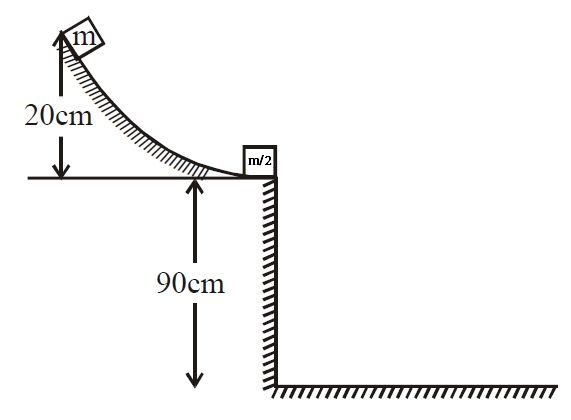# Block's colliding at some height!

In a physics lab, a small cube slides down a friction-less incline as shown in figure, it strikes elastically and horizontally with another cube that is only one-half of its mass. If the incline is $20 cm$ high and the table is $90 cm$ off the floor, big and small cubes strikes the ground at a distance $x$ & $y$ meter respectively from the table . Then $\large \frac{y}{x}$ equal to×